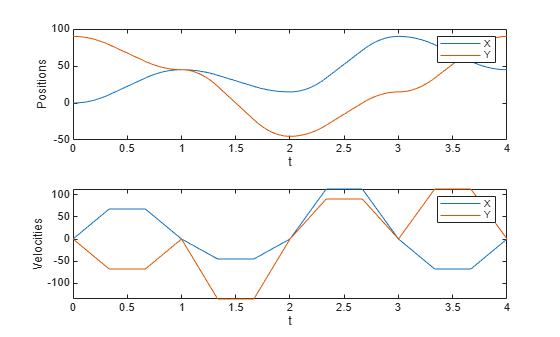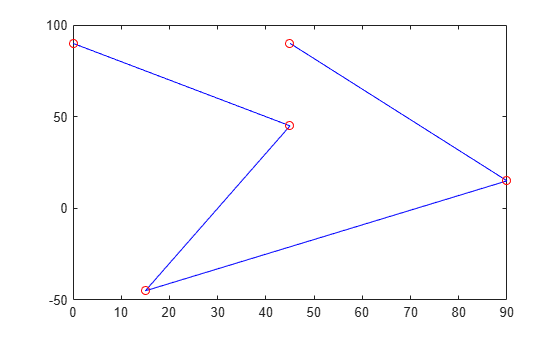Main Content

# trapveltraj

Generate trajectories with trapezoidal velocity profiles

## Syntax

``[q,qd,qdd,tSamples,pp] = trapveltraj(wayPoints,numSamples)``
``[q,qd,qdd,tSamples,pp] = trapveltraj(wayPoints,numSamples,Name,Value)``

## Description

example

````[q,qd,qdd,tSamples,pp] = trapveltraj(wayPoints,numSamples)` generates a trajectory through a given set of input waypoints that follow a trapezoidal velocity profile. The function outputs positions, velocities, and accelerations at the given time samples, `tSamples`, based on the specified number of samples, `numSamples`. The function also returns the piecewise polynomial `pp` form of the polynomial trajectory with respect to time.```
````[q,qd,qdd,tSamples,pp] = trapveltraj(wayPoints,numSamples,Name,Value)` specifies additional parameters using `Name,Value` pair arguments.```

## Examples

collapse all

Use the `trapveltraj` function with a given set of 2-D xy waypoints. Time points for the waypoints are also given.

`wpts = [0 45 15 90 45; 90 45 -45 15 90];`

Compute the trajectory for a given number of samples (501). The function outputs the trajectory positions (`q`), velocity (`qd`), acceleration (`qdd`), time vector (`tvec`), and polynomial coefficients (`pp`) of the polynomial that achieves the waypoints using trapezoidal velocities.

`[q, qd, qdd, tvec, pp] = trapveltraj(wpts, 501);`

Plot the trajectories for the x- and y-positions and the trapezoial velocity profile between each waypoint.

```subplot(2,1,1) plot(tvec, q) xlabel('t') ylabel('Positions') legend('X','Y') subplot(2,1,2) plot(tvec, qd) xlabel('t') ylabel('Velocities') legend('X','Y')```You can also verify the actual positions in the 2-D plane. Plot the separate rows of the `q` vector and the waypoints as x- and y-positions.

```figure plot(q(1,:),q(2,:),'-b',wpts(1,:),wpts(2,:),'or')```## Input Arguments

collapse all

Points for waypoints of trajectory, specified as an n-by-p matrix, where n is the dimension of the trajectory and p is the number of waypoints.

Example: `[1 4 4 3 -2 0; 0 1 2 4 3 1]`

Data Types: `single` | `double`

Number of samples in output trajectory, specified as a positive integer.

Data Types: `single` | `double`

### Name-Value Pair Arguments

Specify optional comma-separated pairs of `Name,Value` arguments. `Name` is the argument name and `Value` is the corresponding value. `Name` must appear inside quotes. You can specify several name and value pair arguments in any order as `Name1,Value1,...,NameN,ValueN`.

Example: `'PeakVelocity',5`

Note

Due to the nature of the trapezoidal velocity profile, you can only set at most two of the following parameters.

Peak velocity of the profile segment, specified as the comma-separated pair consisting of `'PeakVelocity'` and a scalar, vector, or matrix. This peak velocity is the highest velocity achieved during the trapezoidal velocity profile.

A scalar value is applied to all elements of the trajectory and between all waypoints. An n-element vector is applied to each element of the trajectory between all waypoints. An n-by-(p–1) matrix is applied to each element of the trajectory for each waypoint.

Data Types: `single` | `double`

Acceleration of the velocity profile, specified as the comma-separated pair consisting of `'Acceleration'` and a scalar, vector, or matrix. This acceleration defines the constant acceleration from zero velocity to the `PeakVelocity` value.

A scalar value is applied to all elements of the trajectory and between all waypoints. An n-element vector is applied to each element of the trajectory between all waypoints. An n-by-(p–1) matrix is applied to each element of the trajectory for each waypoint.

Data Types: `single` | `double`

Duration of each of the p–1 trajectory segments, specified as the comma-separated pair consisting of `'EndTime'` and a scalar, vector, or matrix.

A scalar value is applied to all elements of the trajectory and between all waypoints. An n-element vector is applied to each element of the trajectory between all waypoints. An n-by-(p–1) matrix is applied to each element of the trajectory for each waypoint.

Data Types: `single` | `double`

Duration of acceleration phase of velocity profile, specified as the comma-separated pair consisting of `'EndTime'` and a scalar, vector, or matrix.

A scalar value is applied to all elements of the trajectory and between all waypoints. An n-element vector is applied to each element of the trajectory between all waypoints. An n-by-(p–1) matrix is applied to each element of the trajectory for each waypoint.

Data Types: `single` | `double`

## Output Arguments

collapse all

Positions of the trajectory at the given time samples in `tSamples`, returned as n-by-m matrix, where n is the dimension of the trajectory, and m is equal to `numSamples`.

Data Types: `single` | `double`

Velocities of the trajectory at the given time samples in `tSamples`, returned as n-by-m matrix, where n is the dimension of the trajectory, and m is equal to `numSamples`.

Data Types: `single` | `double`

Accelerations of the trajectory at the given time samples in `tSamples`, returned as n-by-m matrix, where n is the dimension of the trajectory, and m is equal to `numSamples`.

Data Types: `single` | `double`

Time samples for the trajectory, returned as an m-element vector. The output position, `q`, velocity, `qd`, and accelerations, `qdd` are sampled at these time intervals.

Example: `0:0.01:10`

Data Types: `single` | `double`

Piecewise polynomials, returned as a cell array of structures that defines the polynomial for each section of the piecewise trajectory. If all the elements of the trajectory share the same breaks, the cell array is a single piecewise polynomial structure. Otherwise, the cell array has n elements, which correspond to each of the different trajectory elements (dimensions). Each structure contains the fields:

• `form`: `'pp'`.

• `breaks`: p-element vector of times when the piecewise trajectory changes forms. p is the number of waypoints.

• `coefs`: n(p–1)-by-`order` matrix for the coefficients for the polynomials. n(p–1) is the dimension of the trajectory times the number of `pieces`. Each set of n rows defines the coefficients for the polynomial that described each variable trajectory.

• `pieces`: p–1. The number of breaks minus 1.

• `order`: Degree of the polynomial + 1. For example, cubic polynomials have an order of 4.

• `dim`: n. The dimension of the control point positions.

You can build your own piecewise polynomials using `mkpp`, or evaluate the polynomial at specified times using `ppval`.

Piecewise-polynomial, returned as a structure that defines the polynomial for each section of the piecewise trajectory. You can build your own piecewise polynomials using `mkpp`, or evaluate the polynomial at specified times using `ppval`. The structure contains the fields:

• `form`: `'pp'`.

• `breaks`: p-element vector of times when the piecewise trajectory changes forms. p is the number of waypoints.

• `coefs`: n(p–1)-by-`order` matrix for the coefficients for the polynomials. n(p–1) is the dimension of the trajectory times the number of `pieces`. Each set of n rows defines the coefficients for the polynomial that described each variable trajectory.

• `pieces`: p–1. The number of breaks minus 1.

• `order`: Degree of the polynomial + 1. For example, cubic polynomials have an order of 4.

• `dim`: n. The dimension of the control point positions.

 Lynch, Kevin M., and Frank C. Park. Modern Robotics: Mechanics, Planning and Control. Cambridge: Cambridge University Press, 2017.

 Spong, Mark W., Seth Hutchinson, and M. Vidyasagar. Robot Modeling and Control. John Wiley & Sons, 2006.